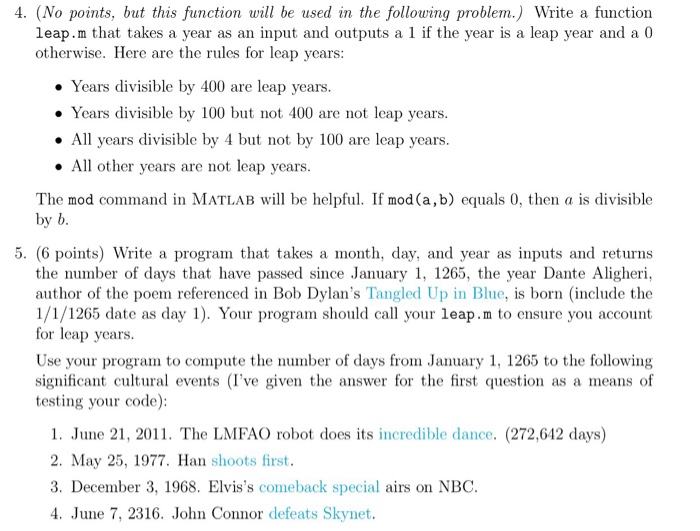# Question & Answer: Write a function leap.m that takes a year as an input and outputs a 1 if the year is a leap year and a 0 otherwise. Here are the rules fo…..Write a function leap.m that takes a year as an input and outputs a 1 if the year is a leap year and a 0 otherwise. Here are the rules for leap years: Years divisible by 400 are leap years. Years divisible by 100 but not 100 are not leap years. All years divisible by 4 but not by 100 are leap years. All other years arc not leap years. The mod command in MATLAB will be helpful. If mod(a, b) equals 0, then a is divisible by b. Write a program that takes a month, day, and year as inputs and returns the number of days that have passed since January 1. 1265, the year Dante Aligheri, author of the poem referenced in Bob Dylan’s Tangled Up in Blue, is born (include the 1/1/1265 date as day 1). Your program should call your leap.m to ensure you account for leap years. Use your program to compute the number of days from January 1, 1265 to the following significant cultural events (I’ve given the answer for the first question as a means of testing your code): June 21, 2011. The LMFAO robot does its incredible dance. (272, 612 days) May 25, 1977. Han shoots first. December 3, 1968. Elvis’s comeback special airs on NBC. June 7, 2316. John Connor defeats Skynet.

function out = leap(year)

Don't use plagiarized sources. Get Your Custom Essay on
Question & Answer: Write a function leap.m that takes a year as an input and outputs a 1 if the year is a leap year and a 0 otherwise. Here are the rules fo…..
GET AN ESSAY WRITTEN FOR YOU FROM AS LOW AS \$13/PAGE

% It returns 0 if the given year is not a leap year,
% It returns 1 if the given year is a leap year.
%
% conditions are:
% years divisible by 400 are leap years
%years divisible by 100 but not 400 are not leap years
%all years divisible by 4 but not by 100 are leap years
all other years are not leap years

out = ( (mod(year,4 ) == 0 ) | (mod(year,400) == 0) ) & ~( (mod(year,100) == 0) & ~(mod(year,400) == 0) );

%year = year(:);
%
%if mod(year,400) == 0
% out = 1;
%else
% if mod(year,100) == 0
% out = 0;
% else
% if mod(year,4) == 0
% out = 1;
% else
% out = 0;
% end;
% end;
%end;